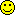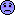Percentage Outcomes using Multinomial...Message/AuthorMike Bader posted on Monday, March 30, 2009 - 8:09 am
I would like to model categorical growth trajectories using a growth mixture modeling approach. My dependent variables are three proportions that cumulatively add to one for each observation (i.e. the proportion group 1 + proportion group 2 + proportion group 3 = 1). Ideally, I would model these using a multinomial distribution since the three proportions always add to one and the proportion of any one group is constrained to be between 0 and 1. Is there a way to model this type of outcome using a multinomial distribution in MPlus? Thank you in advance.Bengt O. Muthen posted on Monday, March 30, 2009 - 10:38 am
Mplus can handle a nominal outcome in combination with latent continuous variables using the multinomial approach that you mention. But you have to think about how the growth model should be understood. With a nominal outcome with 3 categories you model 2 of the 3 categories and unlike ordinal response, those 2 categories can have different predictors, i.e. in principle different growth processes. Are you sure that your outcome should not be treated as ordinal instead of nominal? The ordinal case is straightforward - just declare the outcome as Categorical.Mike Bader posted on Monday, March 30, 2009 - 11:05 am
Dr. Muthen, Thank you very much for your response. I am sure that the outcome is nominal (I am modeling the proportion black, proportion white, and proportion Latino) -- and indeed want to develop trajectories with potentially separate predictors for the two latent growth models (for proportion black and proportion Latino).

Because the outcome variables are proportions of each group (i.e. n_g/N where n_g is the number of each group and N is the total population) rather than a categorical indicator, how would I declare the outcome variables in the VARIABLE and MODEL commands to treat them as multinomially-distributed?

Thank you so much for your help.Tihomir Asparouhov posted on Tuesday, March 31, 2009 - 8:55 am
You can model it as count variables where you will have as dependent variables these two:

NL - number of latinos
NB - number of blacks

NT - the total population will be your exposure constant, so you form the covariate X=log(NT)and by using the commands

NL on X@1;
NB on X@1;

you get that the intercepts [NL] is log of the desired proportion, same for [NB].

To add growth model - simply code it the regular way

i s | NB1 NB2 NB3
i2 s2 | NL1 NL2 NL3

If NT varies across time you will need to construct the time specific exposures X1=Log(NT1) etc...Dimiter  posted on Tuesday, March 31, 2009 - 11:12 am
Dear Tihomir,
(Tiho)
I would like to use the momentum in this discussion and ask a question about the GLM in Mplus when the units of measurement are schools across four school years. For each shool, k, at each year, t, we know the number of students who took a test at year t (N_kt) and the proportion of them who passed the test (P_kt). The task is to run a GLM for the school proportions of success (pass the test), but the problem is how to take into account that these proportions come from different samples for each school at each year (N_kt). One option I was considering was to adjust the proportions by using their weighted logits, where the weigt is: N_kt*P_kt*(1-P_kt), but there might be a better way in Mplus to handle this problem in my GLM scenario.
DimiterTihomir Asparouhov posted on Tuesday, March 31, 2009 - 2:34 pm
Dimiter

I think you can use the same Poisson model with the fixed exposure - as described above.

An alternative model to the Poisson model would be to treat the proportion variables as normal (which is ok when N_kt is not small) and impose the constraint Var(P)=E(P)*(1-E(P))/N_kt
You can do that with model constraints as in example 5.23, but in growth modeling the constraints will become a bit more complicated.Dimiter  posted on Wednesday, April 01, 2009 - 6:25 am
Thanks for the quick response! If I use the Poisson model, shouldn't I use a time specific exposure since N_kt varies across time (school year, t)? This is just for clarification as the alternative model is more appealing to me; (the proportion distributions are pretty much normal in my data). So, I will use the proportion, P_kt, as a dependent variable across time, t, whith the constraint Var(P)=E(P)*(1-E(P))/N_kt, but I may need some more help on the "complications" with this constraints in GLM. I may get back to you in this regard after I try the Mplus run.
DimiterTihomir Asparouhov posted on Wednesday, April 01, 2009 - 10:22 am
Yes, you need the time specific exposure.Mike Bader posted on Wednesday, April 01, 2009 - 11:15 am
Dr. Asparouhov -- I want to thank you for your reply as well, this is very helpful!Dimiter  posted on Thursday, April 02, 2009 - 11:53 am
Dear Tihomir,
I have trouble with runing GLM with the constrint that you suggested, Var(P)=E(P)*(1-E(P))/N_kt. If it is not time consuming, I would appreciate if you can you add the syntax for those constraints in the Mplus progam below (see ???); here X is a background variable, N is the sample size and P is the proportion of students "pass" for each school across 4 time points (years):

DATA: FILE IS "C:\DATA.dat";
FORMAT IS 2F4.0, 4(F6.0, F8.3);
VARIABLE: NAMES ARE SchoolID X N1 P1 N2 P2 N3 P3 N4 P4;
USEVARIABLES ARE X N1 P1 N2 P2 N3 P3 N4 P4;
IDVARIABLE = SchoolID;
CLASSES = c(2);
ANALYSIS: TYPE = MIXTURE;
STARTS 100 10;
MODEL: %OVERALL%
i s| P1 P2 P3 P4; s@0; c#1 ON X; i s ON X;
MODEL CONSTRAINT:

???

OUTPUT: STANDARDIZED RESIDUAL TECH1 TECH11 TECH14;
-----------------------------
Thanks for the help!
DimiterTihomir Asparouhov posted on Monday, April 06, 2009 - 9:56 am
Let me just add a couple of things. The main issue here is called heteroscedasticity, you have observations with variance that varies across observations.

1. If Ni do not vary a lot you should probably ignore this issue.

2. The MLR estimator actually protects against heteroscedasticity so you would get a less efficient estimator with MLR without the constraints but a correct one.

3. You may have a bigger problem than the heteroscedasticity. In a way you are ignoring the measurement error here. If you have small Ni that would be important. You average the tested student results and you are treating this as the observed school average - but that is not the case unless all students have been tested. Entering the students data instead of school averages and using twolevel modeling would resolve that problem and the heteroscedasticity.Tihomir Asparouhov posted on Monday, April 06, 2009 - 9:57 am
Anyway here is how to modify your example (for simplicity just using one class and without covariates) along the lines of example 5.23 to include heteroscedasticity in the model.

DATA: FILE IS "C:\DATA.dat";
FORMAT IS 2F4.0, 4(F6.0, F8.3);
VARIABLE: NAMES ARE SchoolID X N1 P1 N2 P2 N3 P3 N4 P4;
USEVARIABLES ARE P1 P2 P3 P4;
CONSTRAINT = N1 N2 N3 N4;
IDVARIABLE = SchoolID;
MODEL:
i s| P1@0 P2@1 P3@2 P4@3;
[i] (mi);
[s] (ms);
i (vi); s@0;
P1-P4 (v1-v4);

MODEL CONSTRAINT:
v1=mi*(1-mi)/N1-vi;
v2=(mi+ms)*(1-mi-ms)/N2-vi;
v3=(mi+2*ms)*(1-mi-2*ms)/N3-vi;
v4=(mi+3*ms)*(1-mi-3*ms)/N4-vi;Dimiter  posted on Monday, April 06, 2009 - 1:07 pm
Thanks a lot. The M+ code is very helpful. The problem is that the data is provided at school level (i.e., I have no info at the student level). The Ns for a given school vary very little, but they vary a lot across schools.
Keep in touchDimiterDimiter  posted on Wednesday, April 08, 2009 - 9:10 am
Dear Tihomir,
Here is the M+ syntax that you suggested and the error message that I am getting. Any suggestions?

DATA:
FILE IS "C:\DATA.dat";
FORMAT IS F8.0, F4.0, 4(F8.0, F8.3);

VARIABLE:
NAMES ARE SchoolID X N1 P1 N2 P2 N3 P3 N4 P4;
USEVARIABLES ARE P1 P2 P3 P4;
CONSTRAINT = N1 N2 N3 N4;
IDVARIABLE = SchoolID;

MODEL:
i s|P1@0 P2@1 P3@2 P4@3;
[i](mi);
[s](ms);
i(vi);s@0;
P1-P4(v1-v4);

MODEL CONSTRAINT:
v1=mi*(1-mi)/N1-vi;
v2=(mi+ms)*(1-mi-ms)/N2-vi;
v3=(mi+2*ms)*(1-mi-2*ms)/N3-vi;
v4=(mi+3*ms)*(1-mi-3*ms)/N4-vi;

NO CONVERGENCE. SERIOUS PROBLEMS IN ITERATIONS. ESTIMATED COVARIANCE MATRIX NON-INVERTIBLE. CHECK YOUR STARTING VALUES.Linda K. Muthen posted on Wednesday, April 08, 2009 - 4:45 pmTihomir Asparouhov posted on Friday, April 10, 2009 - 4:00 pm
I gave the wrong model constraint statements above. The model constraint statement should have been.

MODEL CONSTRAINT:
NEW(w1 w2 w3 w4);
v1=w1+mi*(1-mi)/N1;
v2=w2+(mi+ms)*(1-mi-ms)/N2;
v3=w3+(mi+2*ms)*(1-mi-2*ms)/N3;
v4=w4+(mi+3*ms)*(1-mi-3*ms)/N4;

Here the interpretation is that wi are the residual variances for the deviation from linear growth model, while the second term accounts for the measurement error in the average.Dimiter  posted on Friday, April 10, 2009 - 6:24 pm
Dear Tiho,
Thanks a lot! Now the program works;
(I wich TECH11 and TECH14 were available under MODEL CONSTRAINTCan you recommend a reference which describes this approach to taking account heteroscedasticity?
��������,
DimiterDimiter  posted on Friday, June 17, 2011 - 11:20 am
Dear Tiho,
Here are the model constraint statements that you provided for a linear growth model of proportions (P1, P2, P3, P4) across 4 time points, given the sample sizes of the proportions (N1, N2, N3, N4):

MODEL CONSTRAINT:
NEW(w1 w2 w3 w4);
v1=w1+mi*(1-mi)/N1;
v2=w2+(mi+ms)*(1-mi-ms)/N2;
v3=w3+(mi+2*ms)*(1-mi-2*ms)/N3;
v4=w4+(mi+3*ms)*(1-mi-3*ms)/N4;

How do you modify this syntax when a quadratic growth model is used?Tihomir Asparouhov posted on Friday, June 17, 2011 - 1:31 pm
Dimiter - try this:

VARIABLE:
USEVARIABLES ARE P1 P2 P3 P4;
CONSTRAINT = N1 N2 N3 N4;

MODEL:
i s q|P1@0 P2@1 P3@2 P4@3;
[i](mi);
[s](ms);
[s](mq);
i(vi);s@0;q@0;
P1-P4(v1-v4);

MODEL CONSTRAINT:
v1=mi*(1-mi)/N1-vi;
v2=(mi+ms+mq)*(1-mi-ms-mq)/N2-vi;
v3=(mi+2*ms+4*mq)*(1-mi-2*ms-4*mq)/N3-vi;
v4=(mi+3*ms+9*mq)*(1-mi-3*ms-9*mq)/N4-vi;Dimiter  posted on Saturday, June 18, 2011 - 11:24 am
Tiho,
I used the model across 5 time points, with the respective adjustments in the statements and the addition of a fifth statement in the model constraint:
v5=(mi+4*ms+16*mq)*(1-mi-4*ms-16*mq)/N5-vi

The error message that I got is:
NO CONVERGENCE. SERIOUS PROBLEMS IN ITERATIONS. ESTIMATED COVARIANCE MATRIX NON-INVERTIBLE. CHECK YOUR STARTING VALUES.

FACTOR SCORES WILL NOT BE COMPUTED DUE TO NONCONVERGENCE OR NONIDENTIFIED MODEL.Tihomir Asparouhov posted on Monday, June 20, 2011 - 1:47 pm
You can probably avoid this problem by properly select starting values for mi, ms, mq, vi. You have to make sure that these starting value yield positive v1,...,v5. If this doesn't work send it to support@statmodel.com.Topics | Tree View | Search | Help/Instructions | Program Credits Administration